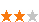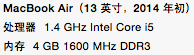您可以捐助，支持我们的公益事业。 1元 10元 50元 认证码：必填求知 文章 文库 Lib 视频 iProcess 课程 认证 咨询 工具 讲座 Modeler Code要资料订阅捐助527 次浏览     评价： 好 中 差
2019-1-14

 编辑推荐: 来源csdn ，文中Python下的各种并发方式，案例说明，介绍较为详细，更多请参考下文。

 from multiprocessing import Process def worker(): """thread worker function""" print'Worker' p=Process(target=worker) p.start() p.join()

Python的mutliprocess模块提供了封装好的管道和队列，可以方便的在进程间传递消息。

Python进程间的同步使用锁，这一点喝线程是一样的。

TCP／IP

TCP／IP是所有远程通信的基础，然而API比较低级别，使用起来比较繁琐，所以一般不会考虑

RPC是早期的远程进程间通信的手段。Python下有一个开源的实现RPyC

Python的开源实现，有许多对远程对象的支持

omniORB （CORBA）

Python下有许多开源的框架来支持分布式的并发，提供有效的管理手段包括：

Celery是一个非常成熟的Python分布式框架，可以在分布式的系统中，异步的执行任务，并提供有效的管理和调度功能。

SCOOP （Scalable COncurrent Operations in Python）提供简单易用的分布式调用接口，使用Future接口来进行并发。

PP （Parallel Python）是另外一个轻量级的Python并行服务

Asyncoro是另一个利用Generator实现分布式并发的Python框架，

greenlet提供轻量级的coroutines来支持进程内的并发。

 from greenlet import greenlet def test1(): print 12 gr2.switch() print34 def test2(): print 56 gr1.switch() print78 gr1=greenlet(test1) gr2=greenlet(test2) gr1.switch()

 12 56 34

eventlet，gevent和concurence都是基于greenlet提供并发的。

eventlet

eventlet是一个提供网络调用并发的Python库，使用者可以以非阻塞的方式来调用阻塞的IO操作。

 import eventlet from eventlet.green import urllib2 urls=['http://www.google.com', 'http://www.example.com', 'http://www.python.org'] def fetch(url): return urllib2.urlopen(url).read() pool=eventlet.GreenPool() for body in pool.imap(fetch,?urls): print("got body", len(body))

 ('got body', 17629) ('got body', 1270) ('got body', 46949)

eventlet为了支持generator的操作对urllib2做了修改，接口和urllib2是一致的。这里的GreenPool和Python的Pool接口一致。

gevent和eventlet类似，关于它们的差异大家可以参考这篇文章

 import gevent from gevent import socket urls = ['www.google.com', 'www.example.com', 'www.python.org'] jobs = [gevent.spawn(socket.gethostbyname, url) for url in urls] gevent.joinall(jobs, timeout=2) print [job.value for job in jobs]

 ['206.169.145.226', '93.184.216.34', '23.235.39.223']

concurence是另外一个利用greenlet提供网络并发的开源库，我没有用过，大家可以自己尝试一下。from math import hypot from random import random def test(tries): return sum(hypot(random(), random()) < 1for_in range(tries))

 from math import hypot from random import random import eventlet import time def test(tries): return sum(hypot(random(), random()) < 1 for _ in range(tries)) def calcPi(nbFutures, tries): ts = time.time() result = map(test, [tries] * nbFutures) ret = 4. * sum(result) / float(nbFutures * tries) span = time.time() - ts print "time spend ", span return ret print calcPi(3000,4000)

 from multiprocessing.dummy import Pool from math import hypot from random import random import time def test(tries): return sum(hypot(random(), random()) < 1 for _ in range(tries)) def calcPi(nbFutures, tries): ts = time.time() p = Pool(1) result = p.map(test, [tries] * nbFutures) ret = 4. * sum(result) / float(nbFutures * tries) span = time.time() - ts print "time spend ", span return ret if __name__ == '__main__': p = Pool() print("pi = {}".format(calcPi(3000, 4000)))from multiprocessing import Pool from math import hypot from random import random import time def test(tries): return sum(hypot(random(), random()) < 1 for _ in range(tries)) def calcPi(nbFutures, tries): ts = time.time() p = Pool(5) result = p.map(test, [tries] * nbFutures) ret = 4. * sum(result) / float(nbFutures * tries) span = time.time() - ts print "time spend ", span return ret if __name__ == '__main__': print("pi = {}".format(calcPi(3000, 4000)))

gevent （伪线程）

 import gevent from math import hypot from random import random import time def test(tries): return sum(hypot(random(), random()) < 1 for _ in range(tries)) def calcPi(nbFutures, tries): ts = time.time() jobs = [gevent.spawn(test, t) for t in [tries] * nbFutures] gevent.joinall(jobs, timeout=2) ret = 4. * sum([job.value for job in jobs]) / float(nbFutures * tries) span = time.time() - ts print "time spend ", span return ret print calcPi(3000,4000)

eventlet （伪线程）

 from math import hypot from random import random import eventlet import time def test(tries): return sum(hypot(random(), random()) < 1 for _ in range(tries)) def calcPi(nbFutures, tries): ts = time.time() pool = eventlet.GreenPool() result = pool.imap(test, [tries] * nbFutures) ret = 4. * sum(result) / float(nbFutures * tries) span = time.time() - ts print "time spend ", span return ret print calcPi(3000,4000)

SCOOP

SCOOP中的Future接口符合PEP-3148的定义，也就是在Python3中提供的Future接口。

 from math import hypot from random import random from scoop import futures import time def test(tries): return sum(hypot(random(), random()) < 1 for _ in range(tries)) def calcPi(nbFutures, tries): ts = time.time() expr = futures.map(test, [tries] * nbFutures) ret = 4. * sum(expr) / float(nbFutures * tries) span = time.time() - ts print "time spend ", span return ret if __name__ == "__main__": print("pi = {}".format(calcPi(3000, 4000)))

Celery

 from celery import Celery from math import hypot from random import random app = Celery('tasks', backend='amqp', broker='amqp://guest@localhost//') app.conf.CELERY_RESULT_BACKEND = 'db+sqlite:///results.sqlite' @app.task def test(tries): return sum(hypot(random(), random()) < 1 for _ in range(tries))

 from celery import group from tasks import test import time def calcPi(nbFutures, tries): ts = time.time() result = group(test.s(tries) for i in xrange(nbFutures))().get() ret = 4. * sum(result) / float(nbFutures * tries) span = time.time() - ts print "time spend ", span return ret print calcPi(3000, 4000)

asyncoro

Asyncoro的测试结果和非并发保持一致。

 import asyncoro from math import hypot from random import random import time def test(tries): yield sum(hypot(random(), random()) < 1 for _ in range(tries)) def calcPi(nbFutures, tries): ts = time.time() coros = [ asyncoro.Coro(test,t) for t in [tries] * nbFutures] ret = 4. * sum([job.value() for job in coros]) / float(nbFutures * tries) span = time.time() - ts print "time spend ", span return ret print calcPi(3000,4000)

IO密集型

IO密集型的任务是另一种常见的用例，例如网络WEB服务器就是一个例子，每秒钟能处理多少个请求时WEB服务器的重要指标。

 from math import hypot import time import urllib2 urls = ['http://www.google.com', 'http://www.example.com', 'http://www.python.org'] def test(url): return urllib2.urlopen(url).read() def testIO(nbFutures): ts = time.time() map(test, urls * nbFutures) span = time.time() - ts print "time spend ", span testIO(10)

Python提供了不同的并发方式，对应于不同的场景，我们需要选择不同的方式进行并发。选择合适的方式，不但要对该方法的原理有所了解，还应该做一些测试和试验，数据才是你做选择的最好参考。527 次浏览  评价: 好 中 差订阅捐助
 相关文章 手机软件测试用例设计实践 手机客户端UI测试分析 iPhone消息推送机制实现与探讨 Android手机开发（一）
 相关文档 Android_UI官方设计教程 手机开发平台介绍 android拍照及上传功能 Android讲义智能手机开发
 相关课程 Android高级移动应用程序 Android系统开发 Android应用开发 手机软件测试